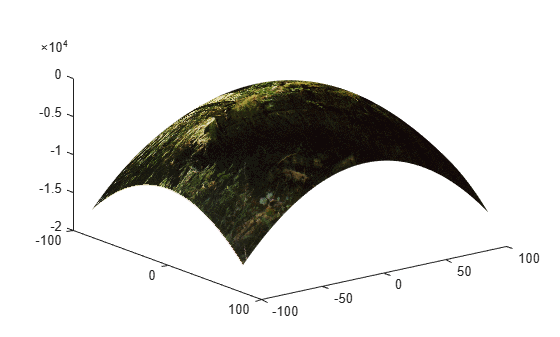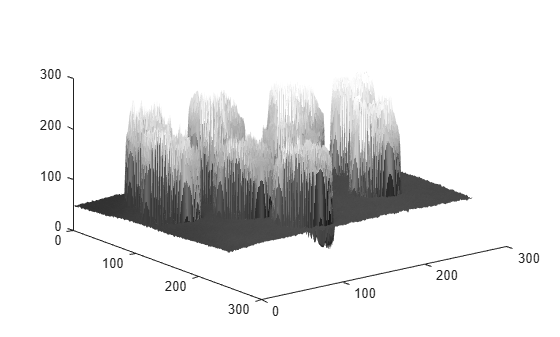warp

Display image as texture-mapped surface

Description

warp(X,map) displays the indexed image X with colormap map as a texture map on a simple rectangular surface.

warp(I,n) displays the intensity image I with n levels as a texture map on a simple rectangular surface.

warp(BW) displays the binary image BW as a texture map on a simple rectangular surface.

warp(RGB) displays the truecolor image RGB as a texture map on a simple rectangular surface.

example

warp(Z,___) displays the image on the surface Z.

example

warp(X,Y,Z,___) displays the image on the surface (X,Y,Z).

h = warp(___) returns a handle to the texture-mapped surface.

Examples

collapse all

This example shows how to warp an indexed image over a nonuniform surface. This example uses a curved surface centered at the origin.

Read an indexed image into the workspace.

Create the surface. First, define the x- and y-coordinates of the surface. This example uses arbitrary coordinates that are unrelated to the indexed image. Note that the size of the coordinate matrices X and Y do not need to match the size of the image.

[X,Y] = meshgrid(-100:100,-80:80);

Define the height Z of the surface at the coordinates given by (X,Y).

Z = -(X.^2 + Y.^2);

Warp the image over the surface defined by the coordinates (X,Y,Z).

figure
warp(X,Y,Z,I,map);Explore the warped image interactively using the rotate and data cursor tools.

Read a grayscale image into the workspace.

Warp the image over the surface whose height is equal to the intensity of the image I. Specify the number of graylevels.

figure
warp(I,I,128);Note that the x- and y-coordinates of the surface were not specified in the call to warp and thus default to the image pixel indices. Explore the warped image interactively using the rotate and data cursor tools.

Input Arguments

collapse all

Indexed image, specified as a 2-D array of real numeric values. The values in X are an index into map, an n-by-3 array of RGB values.

Data Types: single | double | uint8 | uint16 | int16 | logical

Colormap, specified as an n-by-3 array of real numeric values. Each row specifies an RGB color value. When map is type single or double, values must be in the range [0, 1].

Data Types: single | double | uint8

Intensity image, specified as a 2-D array of real numeric values.

Data Types: single | double | uint8 | uint16 | int16 | logical

Number of grayscale levels, specified as a positive integer.

Data Types: double | uint8 | uint16 | logical

Binary image, specified as a 2-D array of logical values.

Data Types: single | double | uint8 | uint16 | int16 | logical

Truecolor image, specified as an m-by-n-by-3 array of real numeric values.

Data Types: single | double | uint8 | uint16 | int16 | logical

Height of surface, specified as a 2-D array of logical values. When Z is not specified, the surface is flat with a uniform height of 0.

Data Types: single | double | uint8 | uint16 | uint32 | uint64 | int8 | int16 | int32 | int64 | logical

x-coordinates of surface, specified as a 2-D array of real numeric values.

Data Types: single | double | uint8 | uint16 | uint32 | uint64 | int8 | int16 | int32 | int64 | logical

y-coordinates of surface, specified as a 2-D array of real numeric values.

Data Types: single | double | uint8 | uint16 | uint32 | uint64 | int8 | int16 | int32 | int64 | logical

Output Arguments

collapse all

Texture-mapped surface object created by warp, specified as a texture-mapped surface object.

Tips

• Texture-mapped surfaces are generally rendered more slowly than images.